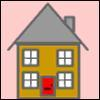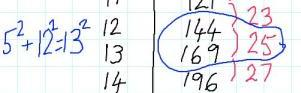#### You may also like### Triangular Triples

Show that 8778, 10296 and 13530 are three triangular numbers and that they form a Pythagorean triple.### Iff### Smith and Jones

Mr Smith and Mr Jones are two maths teachers. By asking questions, the answers to which may be right or wrong, Mr Jones is able to find the number of the house Mr Smith lives in... Or not!

# Generating Triples

##### Age 14 to 16 Challenge Level:
Here is an extract from Charlie's table:Where is the $5^2$?
Where is the $12^2$?
Where is the $13^2$?

Where might we find a similar set of related square numbers?